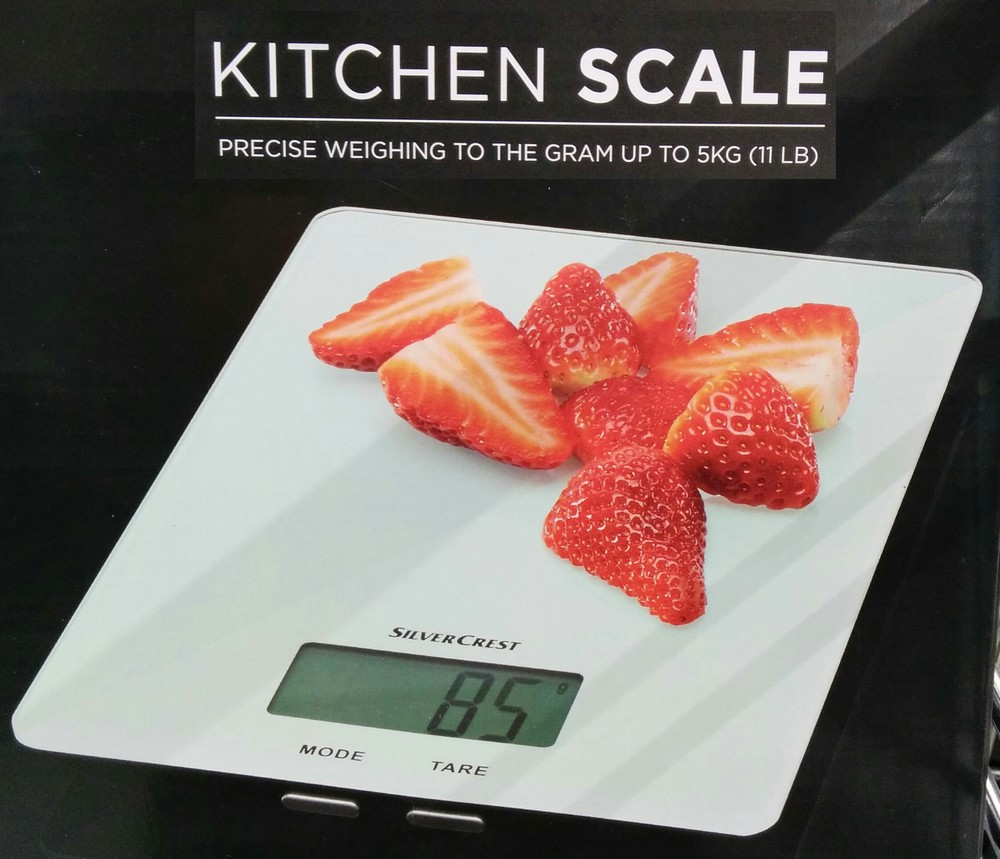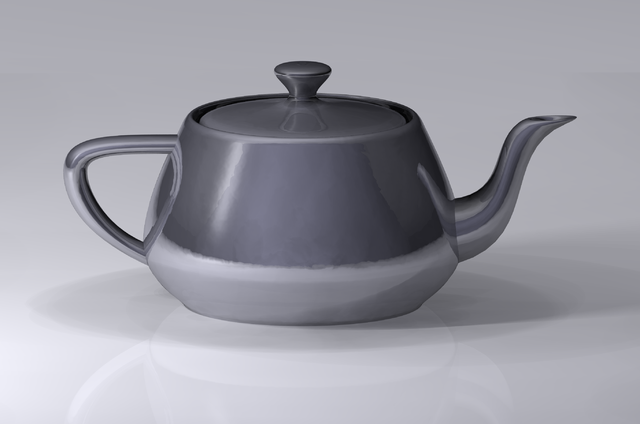# Viz - Scale

Scale in Visualization

• Line with numbers (Major and minor Grid)

Scale map:

• data value (a domain)
• to pixels value (a range).

Scale permits data (the domain) to fit the chart area (the pixels range).

## Domain - Range Mapping

### Javascript

• Scale x
``````MAX_X = 10; // Max value
WIDTH = 10; // Max Length / color
let x = ( val => val / MAX_X * WIDTH );```
```
• Scale Y
``````MAX_Y = 10; // Max Value
HEIGHT = 20; // Max Length / color
// The scale is negative here (goes from 10 to 0)
let y = ( val => HEIGHT - val / MAX_Y * HEIGHT );```
```
• Example Output
``````console.log("X");
console.log("  * at 0: "+x(0));
console.log("  * at 5: "+x(5));
console.log("  * at 10: "+x(10));
console.log("Y: ");
console.log("  * at 0: "+y(0));
console.log("  * at 5: "+y(5));
console.log("  * at 10: "+y(10));```
```

### d3

Same in D3 - Scale

``````let x = scaleLinear().domain([0, MAX_X]).range([0, WIDTH]);
let y = scaleLinear().domain([0, MAX_Y]).range([HEIGHT, 0]);```
```

Discover MoreD3 - Scale

scale in D3. D3’s scales simplify visual encoding. They map data value (a domain) to pixels value (a range). These scales supports both ordinal and quantitative (linear, logarithmic, exponential,...Statistics - Scale

Scale Or unit of variable, coefficient: m km ... from 0->inf into 0->1: See mmalex/status/916313043767906304?s=03tanh(mmalex) seeViz - Legend

pysal/legendgram color scale shown as a distribution